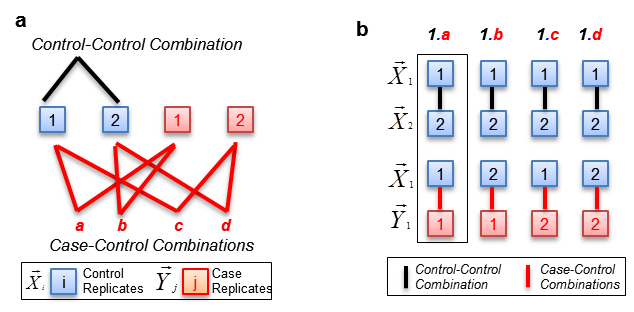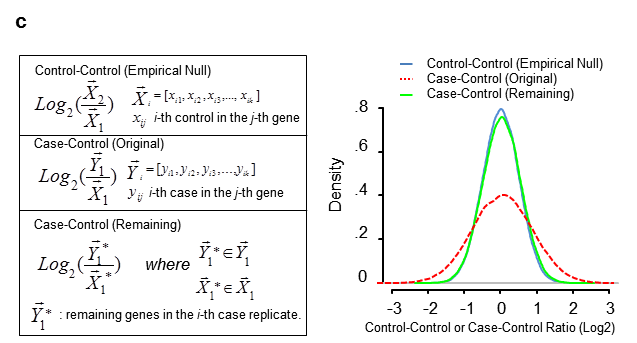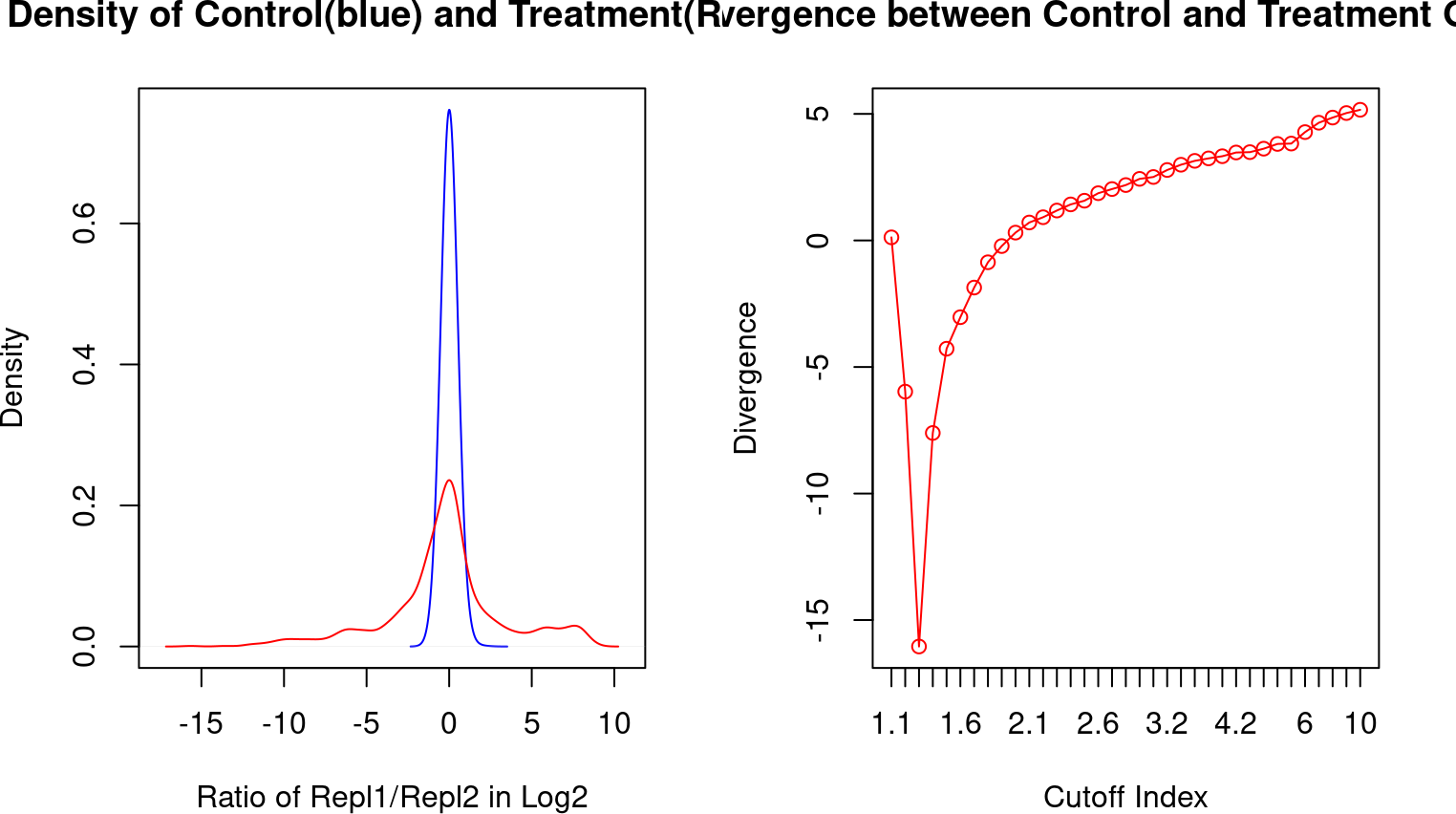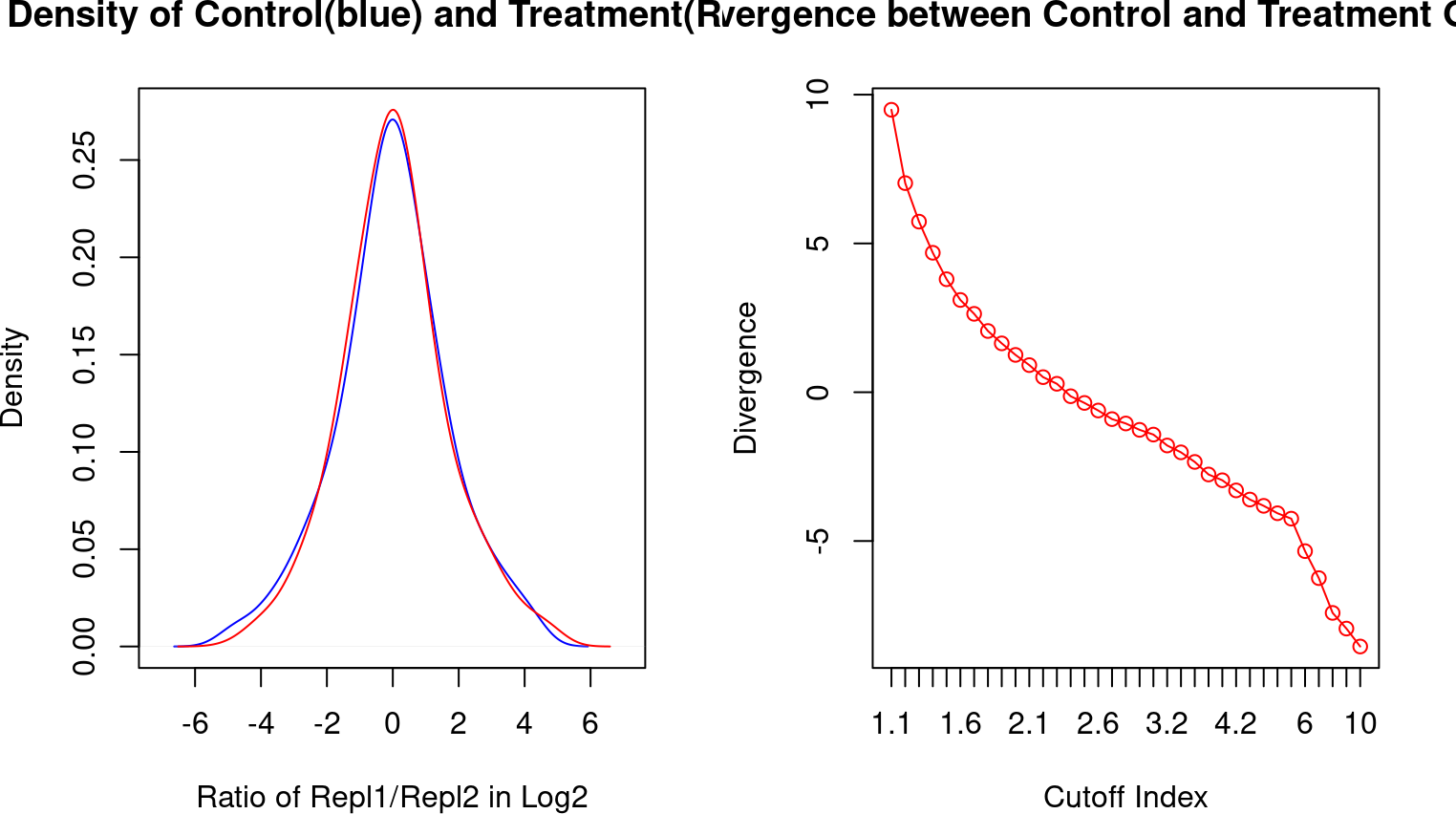# 1 Introduction to fCI

## 1.1 Authors and Affliations

Shaojun Tang1, Martin Hemberg2, Ertugrul Cansizoglu3, Stephane Belin3, Kenneth Kosik4, Gabriel Kreiman2, Hanno Steen1#+, Judith Steen3#+

1Departments of Pathology, Boston Childrens Hospital and Harvard Medical School, Boston, MA, USA, 02115

2Department of Ophthalmology, Boston Childrens Hospital, Boston, MA, USA, 02115

3F.M. Kirby Neurobiology Center, Childrens Hospital, and Department of Neurology, Harvard Medical School, 300 Longwood Avenue, Boston, MA, USA, 02115

4Neuroscience Research Institute, University of California at Santa Barbara, Santa Barbara, CA, USA, 93106"

## 1.2 Abstract

"The ability to integrate ‘omics’ (i.e., transcriptomics and proteomics) is becoming increasingly important to understanding regulatory mechanisms. There are currently no tools available to identify differentially expressed genes (DEGs) across different ‘omics’ data types or multi-dimensional data including time courses. We present a model capable of simultaneously identifying DEGs from continuous and discrete transcriptomic, proteomic and integrated proteogenomic data. We show that our algorithm can be used across multiple diverse sets of data and can unambiguously find genes that show functional modulation, developmental changes or misregulation. Applying our model to a time course proteogenomics dataset, we identified a number of important genes that showed distinctive regulation patterns.

## 1.3 Introduction

fCI (f-divergence Cutoff Index), identifies DEGs by computing the difference between the distribution of fold-changes for the control-control and remaining (non-differential) case-control gene expression ratio data.As a null hypothesis, we assume that the control samples, regardless of data types, do not contain DEGs and that the spread of the control data reflects the biological and technical variance in the data. In contrast, the case samples contain a yet unknown number of DEGs. Removing DEGs from the case data leaves a set of non-differentially expressed genes whose distribution is identical to the control samples. Our method, f-divergence cut-out index (fCI) identifies DEGs by computing the difference between the distribution of fold-changes for the control-control data and remaining (non-differential) case-control gene expression ratio data (see Fig. 1.a-b) upon removal of genes with large fold changesfCI provides several advantages compared to existing methods. Firstly, it performed equally well or better in finding DEGs in diverse data types (both discrete and continuous data) from various omics technologies compared to methods that were specifically designed for the experiments. Secondly, it fulfills an urgent need in the omics research arena. The increasingly common proteogenomic approaches enabled by rapidly decreasing sequencing costs facilitates the collection of multi-dimensional (i.e. proteogenomics) experiments, for which no efficient tools have been developed to find co-regulation and dependences of DEGs between treatment conditions or developmental stages. Thirdly, fCI does not rely on statistical methods that require sufficiently large numbers of replicates to evaluate DEGs. Instead fCI can effectively identify changes in samples with very few or no replicates.

# 2 Installing fCI

fCI should be installed as follows:

``````if (!requireNamespace("BiocManager", quietly=TRUE))
install.packages("BiocManager")
BiocManager::install("fCI")``````
`````` suppressPackageStartupMessages(library(fCI))
library(fCI)``````

# 3 Differential Expression Analysis using fCI

fCI is very usefriendly. Users only need to provide a ‘Tab’ delimited input data file and give the indexes of control and case samples.

## 3.1 Reading the input data:

Read Inupt Data to R** . This input will contain gene, protein or other expression values with columns representing samples/lanes/replicates, and rows representing genes.

### 3.1.1 Integer raw read counts from NGS data or Spectrum counts from proteomics data

As input, the fCI package could analysis count data as obtained, e. g., from RNA-seq or another high-throughput sequencing experiment, in the form of a matrix of integer values. The value in the i-th row and the j-th column of the matrix tells how many reads have been mapped to gene i in sample j. Analogously, for other types of assays, the rows of the matrix might correspond e. g. to binding regions (with ChIP-Seq) or peptide sequences (with quantitative mass spectrometry).

### 3.1.2 Normalized gene expression such as RPKM or FPKM, or peak intesntiy (height/area) in proteomics data

The fCI package could also analyze decimal data in the form of RPKM/FPKM from RNA-seq or another high-throughput sequencing experiment, in the form of a matrix of integer values. The value in the i-th row and the j-th column of the matrix tells the normalized expression level in gene i and sample j.

### 3.1.3 Ratio data from many experiments measuring relative gene expression with respect to control channels.

For example, relative protein quantification by MS/MS using the tandem mass tag technology are represented by ratios.

## 3.2 Data normalization

### 3.2.1 Total library normalization

The samples are normalized to have the same library size (i.e. total raw read counts) if the experiment replicates were obtained by the same protocol and an equal library size was expected within each experimental condition. The fCI will apply the sum normalization so that each column has equal value by summing all the genes of each replicate.

``````fci.data=data.frame(matrix(sample(3:100, 1043*6, replace=TRUE), 1043,6))
fci.data=total.library.size.normalization(fci.data)``````

### 3.2.2 Trimed sum normalization

We could normalize each replicate to have the same library size (total read count) after the 5% lowly expressed and the 5% highly expressed genes were removed from each replicate

``````fci.data=data.frame(matrix(sample(3:100, 1043*6, replace=TRUE), 1043,6))
fci.data=trim.size.normalization(fci.data)``````

### 3.2.3 Kernel density distribution centering

We hypothesized that the genes whose expression was the least affected by the experiment (in the forms of both RNA and protein) should have nearly identical expression levels across different replicates, in both RNA-Seq and proteomic datasets. These unchanged genes will be centered at zero in the logarithm transformed control-control or case-control ratio distributions. Therefore, we normalized proteogenomic dataset’s fCI pairwise ratio distribution (Gaussian kernel density approximation) to be centered at zero.

## 3.3 fCI analysis with the Spike-in microarray data

• The Spike-in data contained a number of spiked-in differentially expressed genes with a known cutoff of 1.4 fold threshold.

• The input data is a tab-delimited file with rows representing genes and columns being the samples of control and experimental treatments. T

• To find the DEGs, we first created a fCI class object named fci, which will be passed onto the main function “find.fci.targets”. In the function call, the users need to specify the control sample column ids (such as a vector of 1, 2 and 3) and case sample column ids (such as a vector of 4, 5 and 6). Each sample must contain the same number of genes.

• For the chosen control samples, fCI forms a list of the control-control combinations, namely 1-2, 1-3 and 2-3, each containing two unique replicates from the full set of control replicates. Similarly, fCI forms a list of control-case combinations, namely, 1-4, 1-5, 1-6, 2-4, 2-5, 2-6, 3-4, 3-5 and 3-6, each containing a unique replicate from the control and a unique replicate from the case samples.

``````pkg.path=path.package('fCI')
filename=paste(pkg.path, "/extdata/Supp_Dataset_part_2.txt", sep="")

if(file.exists(filename)){
fci=new("NPCI")
fci=find.fci.targets(fci, c(1,2,3), c(4,5,6), filename)
}``````
``````## Control-Control Used : [ 1 2 ] & Control-Case Used : [ 1 4 ];  Fold_Cutoff= 1.3 ; Num_Of_DEGs= 821 ; Divergence= 0.00015769
## Control-Control Used : [ 1 2 ] & Control-Case Used : [ 2 4 ];  Fold_Cutoff= 1.3 ; Num_Of_DEGs= 811 ; Divergence= 0.00069831
## Control-Control Used : [ 1 2 ] & Control-Case Used : [ 3 4 ];  Fold_Cutoff= 1.3 ; Num_Of_DEGs= 813 ; Divergence= 0.00021425
## Control-Control Used : [ 1 2 ] & Control-Case Used : [ 1 5 ];  Fold_Cutoff= 1.3 ; Num_Of_DEGs= 820 ; Divergence= 0.00056408
## Control-Control Used : [ 1 2 ] & Control-Case Used : [ 2 5 ];  Fold_Cutoff= 1.3 ; Num_Of_DEGs= 819 ; Divergence= 0.00010632
## Control-Control Used : [ 1 2 ] & Control-Case Used : [ 3 5 ];  Fold_Cutoff= 1.3 ; Num_Of_DEGs= 820 ; Divergence= 0.00057505
## Control-Control Used : [ 1 2 ] & Control-Case Used : [ 1 6 ];  Fold_Cutoff= 1.3 ; Num_Of_DEGs= 823 ; Divergence= 0.00023359
## Control-Control Used : [ 1 2 ] & Control-Case Used : [ 2 6 ];  Fold_Cutoff= 1.3 ; Num_Of_DEGs= 813 ; Divergence= 0.00113844
## Control-Control Used : [ 1 2 ] & Control-Case Used : [ 3 6 ];  Fold_Cutoff= 1.3 ; Num_Of_DEGs= 813 ; Divergence= 0.00036187
## Control-Control Used : [ 1 3 ] & Control-Case Used : [ 1 4 ];  Fold_Cutoff= 1.3 ; Num_Of_DEGs= 821 ; Divergence= 1.764e-05
## Control-Control Used : [ 1 3 ] & Control-Case Used : [ 2 4 ];  Fold_Cutoff= 1.3 ; Num_Of_DEGs= 811 ; Divergence= 0.00014706
## Control-Control Used : [ 1 3 ] & Control-Case Used : [ 3 4 ];  Fold_Cutoff= 1.3 ; Num_Of_DEGs= 813 ; Divergence= 1.477e-05
## Control-Control Used : [ 1 3 ] & Control-Case Used : [ 1 5 ];  Fold_Cutoff= 1.3 ; Num_Of_DEGs= 820 ; Divergence= 0.00014493
## Control-Control Used : [ 1 3 ] & Control-Case Used : [ 2 5 ];  Fold_Cutoff= 1.3 ; Num_Of_DEGs= 819 ; Divergence= 2.89e-06
## Control-Control Used : [ 1 3 ] & Control-Case Used : [ 3 5 ];  Fold_Cutoff= 1.3 ; Num_Of_DEGs= 820 ; Divergence= 0.00012726
## Control-Control Used : [ 1 3 ] & Control-Case Used : [ 1 6 ];  Fold_Cutoff= 1.3 ; Num_Of_DEGs= 823 ; Divergence= 5.034e-05
## Control-Control Used : [ 1 3 ] & Control-Case Used : [ 2 6 ];  Fold_Cutoff= 1.3 ; Num_Of_DEGs= 813 ; Divergence= 0.00024359
## Control-Control Used : [ 1 3 ] & Control-Case Used : [ 3 6 ];  Fold_Cutoff= 1.3 ; Num_Of_DEGs= 813 ; Divergence= 3.525e-05
## Control-Control Used : [ 2 3 ] & Control-Case Used : [ 1 4 ];  Fold_Cutoff= 1.3 ; Num_Of_DEGs= 821 ; Divergence= 2e-08
## Control-Control Used : [ 2 3 ] & Control-Case Used : [ 2 4 ];  Fold_Cutoff= 1.3 ; Num_Of_DEGs= 811 ; Divergence= 1.509e-05
## Control-Control Used : [ 2 3 ] & Control-Case Used : [ 3 4 ];  Fold_Cutoff= 1.3 ; Num_Of_DEGs= 813 ; Divergence= 2.82e-06
## Control-Control Used : [ 2 3 ] & Control-Case Used : [ 1 5 ];  Fold_Cutoff= 1.3 ; Num_Of_DEGs= 820 ; Divergence= 8.66e-06
## Control-Control Used : [ 2 3 ] & Control-Case Used : [ 2 5 ];  Fold_Cutoff= 1.3 ; Num_Of_DEGs= 819 ; Divergence= 2.82e-06
## Control-Control Used : [ 2 3 ] & Control-Case Used : [ 3 5 ];  Fold_Cutoff= 1.3 ; Num_Of_DEGs= 820 ; Divergence= 8.36e-06
## Control-Control Used : [ 2 3 ] & Control-Case Used : [ 1 6 ];  Fold_Cutoff= 1.3 ; Num_Of_DEGs= 823 ; Divergence= 5.8e-07
## Control-Control Used : [ 2 3 ] & Control-Case Used : [ 2 6 ];  Fold_Cutoff= 1.3 ; Num_Of_DEGs= 813 ; Divergence= 6.742e-05
## Control-Control Used : [ 2 3 ] & Control-Case Used : [ 3 6 ];  Fold_Cutoff= 1.3 ; Num_Of_DEGs= 813 ; Divergence= 1.48e-05``````

## 3.4 fCI DEG analysis Output

• the returned object ‘fci’ will contains all the differentially expressed genes and runtime variables, including the DEGs and kernel density distributions.

### 3.4.2 The Kernel Density Plot of Control-Control and Control-Case distributions

``  figures(fci)``
``##  426.0178``• The kernel density plot shows the distribution of logarithm ratios in the control-control dataset and case-control dataset. In general, the control- control distribution should reflects the system noise while the case-control will contains real DEGs and system noises.

• Instead of using all control and case samples, the user could specify a small
sample and perform a pilot study. This is extremely useful if the users are only interested on a small subset of samples.

``  fci=find.fci.targets(fci, c(1,2), 5, filename)``
``````## Control-Control Used : [ 1 2 ] & Control-Case Used : [ 1 5 ];  Fold_Cutoff= 1.3 ; Num_Of_DEGs= 820 ; Divergence= 0.00056408
## Control-Control Used : [ 1 2 ] & Control-Case Used : [ 2 5 ];  Fold_Cutoff= 1.3 ; Num_Of_DEGs= 819 ; Divergence= 0.00010632``````

## 3.5 Alternative function to find DEGs

• Using the same microarray dataset, we could run fCI with a single function call. However, the internal runtime variables and fci object will disappear after the function returns.
``````if(file.exists(filename)){
Diff.Expr.Genes=fCI.call.by.index(c(1,2,3), c(4,5,6), filename)
}``````
``````## Control-Control Used : [ 1 2 ] & Control-Case Used : [ 1 4 ];  Fold_Cutoff= 1.3 ; Num_Of_DEGs= 821 ; Divergence= 0.00015769
## Control-Control Used : [ 1 2 ] & Control-Case Used : [ 2 4 ];  Fold_Cutoff= 1.3 ; Num_Of_DEGs= 811 ; Divergence= 0.00069831
## Control-Control Used : [ 1 2 ] & Control-Case Used : [ 3 4 ];  Fold_Cutoff= 1.3 ; Num_Of_DEGs= 813 ; Divergence= 0.00021425
## Control-Control Used : [ 1 2 ] & Control-Case Used : [ 1 5 ];  Fold_Cutoff= 1.3 ; Num_Of_DEGs= 820 ; Divergence= 0.00056408
## Control-Control Used : [ 1 2 ] & Control-Case Used : [ 2 5 ];  Fold_Cutoff= 1.3 ; Num_Of_DEGs= 819 ; Divergence= 0.00010632
## Control-Control Used : [ 1 2 ] & Control-Case Used : [ 3 5 ];  Fold_Cutoff= 1.3 ; Num_Of_DEGs= 820 ; Divergence= 0.00057505
## Control-Control Used : [ 1 2 ] & Control-Case Used : [ 1 6 ];  Fold_Cutoff= 1.3 ; Num_Of_DEGs= 823 ; Divergence= 0.00023359
## Control-Control Used : [ 1 2 ] & Control-Case Used : [ 2 6 ];  Fold_Cutoff= 1.3 ; Num_Of_DEGs= 813 ; Divergence= 0.00113844
## Control-Control Used : [ 1 2 ] & Control-Case Used : [ 3 6 ];  Fold_Cutoff= 1.3 ; Num_Of_DEGs= 813 ; Divergence= 0.00036187
## Control-Control Used : [ 1 3 ] & Control-Case Used : [ 1 4 ];  Fold_Cutoff= 1.3 ; Num_Of_DEGs= 821 ; Divergence= 1.764e-05
## Control-Control Used : [ 1 3 ] & Control-Case Used : [ 2 4 ];  Fold_Cutoff= 1.3 ; Num_Of_DEGs= 811 ; Divergence= 0.00014706
## Control-Control Used : [ 1 3 ] & Control-Case Used : [ 3 4 ];  Fold_Cutoff= 1.3 ; Num_Of_DEGs= 813 ; Divergence= 1.477e-05
## Control-Control Used : [ 1 3 ] & Control-Case Used : [ 1 5 ];  Fold_Cutoff= 1.3 ; Num_Of_DEGs= 820 ; Divergence= 0.00014493
## Control-Control Used : [ 1 3 ] & Control-Case Used : [ 2 5 ];  Fold_Cutoff= 1.3 ; Num_Of_DEGs= 819 ; Divergence= 2.89e-06
## Control-Control Used : [ 1 3 ] & Control-Case Used : [ 3 5 ];  Fold_Cutoff= 1.3 ; Num_Of_DEGs= 820 ; Divergence= 0.00012726
## Control-Control Used : [ 1 3 ] & Control-Case Used : [ 1 6 ];  Fold_Cutoff= 1.3 ; Num_Of_DEGs= 823 ; Divergence= 5.034e-05
## Control-Control Used : [ 1 3 ] & Control-Case Used : [ 2 6 ];  Fold_Cutoff= 1.3 ; Num_Of_DEGs= 813 ; Divergence= 0.00024359
## Control-Control Used : [ 1 3 ] & Control-Case Used : [ 3 6 ];  Fold_Cutoff= 1.3 ; Num_Of_DEGs= 813 ; Divergence= 3.525e-05
## Control-Control Used : [ 2 3 ] & Control-Case Used : [ 1 4 ];  Fold_Cutoff= 1.3 ; Num_Of_DEGs= 821 ; Divergence= 2e-08
## Control-Control Used : [ 2 3 ] & Control-Case Used : [ 2 4 ];  Fold_Cutoff= 1.3 ; Num_Of_DEGs= 811 ; Divergence= 1.509e-05
## Control-Control Used : [ 2 3 ] & Control-Case Used : [ 3 4 ];  Fold_Cutoff= 1.3 ; Num_Of_DEGs= 813 ; Divergence= 2.82e-06
## Control-Control Used : [ 2 3 ] & Control-Case Used : [ 1 5 ];  Fold_Cutoff= 1.3 ; Num_Of_DEGs= 820 ; Divergence= 8.66e-06
## Control-Control Used : [ 2 3 ] & Control-Case Used : [ 2 5 ];  Fold_Cutoff= 1.3 ; Num_Of_DEGs= 819 ; Divergence= 2.82e-06
## Control-Control Used : [ 2 3 ] & Control-Case Used : [ 3 5 ];  Fold_Cutoff= 1.3 ; Num_Of_DEGs= 820 ; Divergence= 8.36e-06
## Control-Control Used : [ 2 3 ] & Control-Case Used : [ 1 6 ];  Fold_Cutoff= 1.3 ; Num_Of_DEGs= 823 ; Divergence= 5.8e-07
## Control-Control Used : [ 2 3 ] & Control-Case Used : [ 2 6 ];  Fold_Cutoff= 1.3 ; Num_Of_DEGs= 813 ; Divergence= 6.742e-05
## Control-Control Used : [ 2 3 ] & Control-Case Used : [ 3 6 ];  Fold_Cutoff= 1.3 ; Num_Of_DEGs= 813 ; Divergence= 1.48e-05``````
``````##   DEG_Names Mean_Control Mean_Case Log2_FC fCI_Prob_Score
## 1         1        0.009     0.002   -2.17              1
## 2        10        0.672     0.091  -2.885              1
## 3       100        1.266     0.399  -1.666              1
## 4      1000        0.239     0.456   0.932              1
## 5      1001        0.136     0.357   1.392              1
## 6      1002        0.004     0.014   1.807              1``````

## 3.6 Testing fCI on a randomly generated simulated dataset

• In this example, we generated a simulated random dataset with 3 control replicates (column 1 through 3) and 3 case replicates (columns 4 through 6).
``fci.data=data.frame(matrix(sample(3:100, 1043*6, replace=TRUE), 1043,6))``

### 3.6.1 Finding Differentially Expressed Genes (no DEGs in this case):

• To identify differentially expressed genes using the above simulated data:
`````` library(fCI)
fci=new("NPCI")
targets=find.fci.targets(fci, c(1,2,3), c(4,5,6), fci.data)
Diff.Expr.Genes=show.targets(targets)``````
``##  "No differentially expressed genes are found!"``
`` head(Diff.Expr.Genes)``
``## NULL``
`` figures(targets)``
``##  34.06239``• fCI didn’t find a local minimum divergence under the given cutoff fold changes. This confirms that there is indeed no differentially expressed genes.

• This analysis strongly proved that fCI is able to distinguish real DEGs from system noise. If the distribution of case-control didn’t show obivous deviation from control-control, no DEGs will be reported.

# 4 Multi-dimensional (i.e.Pproteogenomics data) fCI analysis

• If the dataset contains multiple control replicates and case replicates, the users don’t need to form the these combinations and perform fCI individually. Instead, users could invoke fci on a top-level function that will automatically perform the analysis on given control replicate column ids and case/experimental column ids.

Formation of empirical & experimental distributions on integrated and/or multidimensional (i.e. time course data). In this example, gene expression values are recorded at c dimensions (c=2 in this figure) with m replicates at each condition from a total of n genes. The ratio of the chosen fCI control-control (or control-case) on 2-dimensional measurements will undergo logarithm transformation and normalization for the analysis. If the pathological or experimental condition causes a number of genes to be up-regulated or down-regulated, a wider distribution which can be described by kernel density distribution (indicated by the 3D ellipse in red) compared to the control-control empirical null distribution (indicated by the 3D ellipse in blue) will be observed. fCI then gradually removes the genes from both tails (representing genes having larger fold changes) from both dimensions using the Hellinger Divergence or Cross Entropy estimation (see methods and materials) until the remaining case-control distribution is very similar or identical to the empirical null distribution, as indicated by the kern density distribution